• fmath-mathml-java-latex-mathml.jar.rar中包含3个jar，具体如下： fmath-latex-mathml-0.5.jar fmath-mathml-1.0.jar fmath-mathml-java-3.1.jar
• 在 Linux、macOS、Windows 系统下使用 Mathpix 可以截取到复杂数学方程式的截图，并可以转换成 LaTeX 可编辑文本，在写学术及科学文献时经常会用到。编写 LaTeX 文档最麻烦的部分是那些复杂的方程式，使用 Mathpix ...
• math.h just great,if you use this,don't shout it,please.c语言
• math3是一款非常好用的工具，里面提供了各种运算的方法及类，方便大家调用。
• 1.Random()、Math.random()、Math.round()函数的区别;1.Random()、Math.random()、Math.round()函数的区别;1.Random()、Math.random()、Math.round()函数的区别;1）Random()：创建一个新的随机数生成器。此构造方法...
1.Random()、Math.random()、Math.round()函数的区别;1.Random()、Math.random()、Math.round()函数的区别;1.Random()、Math.random()、Math.round()函数的区别;

1）Random()：创建一个新的随机数生成器。此构造方法将随机数生成器的种子设置为某个值，该值与此构造方法的所有其他调用所用的值完全不同。

Random(long seed)使用单个long 种子创建一个新的随机数生成器。该种子是伪随机数生成器的内部状态的初始值，该生成器可通过方法 next(int) 维护。

Random 的一个特点是：相同种子数的Random对象，对应相同次数生成的随机数字是完全相同的；

Random random1 = new Random(10);

Random random2 = new Random(10);

random1、random2 对应相同次数生成的随机数字完全相同。比如：

System.out.println(ran.nextInt(10));

System.out.print(ran2.nextInt(10));

输出结果：

5

5

2）Math.random()能够返回带正号的double值，该值大于等于0.0且小于1.0，即取值范围是[0.0,1.0)的左闭右开区间，返回值是一个伪随机选择的数，在该范围内（近似）均匀分布。

for(int i = 0; i < 10; i++ ){

System.out.println((int)(Math.random()*5));

}

输出结果：

4，2，0，1，3，3，4，0，3，3

在使用Math.Random()的时候，需要注意的地方是该函数是返回double类型的值，所以在要赋值给其他类型的变量的时候注意需要进行塑形转换。
3）（四舍五入）Math.round(float a)返回最接近参数的 int;
Math.round(double a)返回最接近参数的 long。
System.out.println(Math.round(1.5));
System.out.println(Math.round(-1.5));
System.out.println(Math.round(0.5));
System.out.println(Math.round(-0.5));
输出：

2

-1

1

0
特殊情况如下：

如果参数为 NaN，那么结果为 0。

如果结果为负无穷大或任何小于等于 Integer.MIN_VALUE 的值，那么结果等于 Integer.MIN_VALUE 的值。

如果参数为正无穷大或任何大于等于 Integer.MAX_VALUE 的值，那么结果等于 Integer.MAX_VALUE 的值。

（向上取整）Math.ceil(double a)返回最小的（最接近负无穷大） double 值，该值大于等于参数，并等于某个整数。特殊情况如下：

如果参数值已经等于某个整数，那么结果与该参数相同。

如果参数为 NaN、无穷大、正 0 或负 0，那么结果与参数相同。

如果参数值小于 0，但是大于 -1.0，那么结果为负 0。
System.out.println(Math.ceil(1.2));
System.out.println(Math.ceil(-1.2));
System.out.println(Math.ceil(0.6));
System.out.println(Math.ceil(-0.6));
输出：

2.0

-1.0

1.0

-0.0-0.0-0.0

展开全文Random()
• ## Math

千次阅读 2015-12-21 23:21:55
Math
java.math.Math类常用的常量和方法：
Math.PI 记录的圆周率
Math.E记录e的常量
Math.abs 求绝对值
Math.sin 正弦函数 Math.asin 反正弦函数
Math.cos 余弦函数 Math.acos 反余弦函数
Math.tan 正切函数 Math.atan 反正切函数&nbsp;Math.atan2 商的反正切函数
Math.ceil 得到不小于某数的最大整数
Math.floor 得到不大于某数的最大整数
例如：Math.floor(12.7) =12.0  Math.ceil(12.7) =13.0  ceil()是天花板，即向上取整。floor是地板，向下取整。round是四舍五入。
Math.IEEEremainder 求余
Math.max 求两数中最大
Math.min 求两数中最小
Math.sqrt 求开方
Math.pow 求某数的任意次方, 抛出ArithmeticException处理溢出异常
Math.sqrt(x)：平方根
Math.pow(x,y)：x的y次方
Math.exp 求e的任意次方
Math.log10 以10为底的对数
Math.log 自然对数
Math.rint 求距离某数最近的整数（可能比某数大，也可能比它小）
Math.round 同上，返回int型或者long型（上一个函数返回double型）
Math.random 返回0，1之间的一个随机数
java.math.BigInteger(大整数)：
BigInteger bi1=new BigInteger("1234567890123456890");
BigInteger bi2=BigInteger.valueOf(123L);
bi1=bi1.multiply(bi2);//b1*b2
bi1=bi1.subtract(bi2);//b1-b2
bi1=bi1.divide(bi2);// b1/b2
java.math.BigDecimal(大浮点数):
BigDecimal bd = new BigDecimal("3.1415926");
bd = bd.setScale(2,BigDecimal.ROUND_DOWN);//取3.1415926小数点后面二位
展开全文• 缺少这个jar包的或者缺少commons-math2.2.jar的小伙伴们可以来用这个jar包啦
• ## c语言库函数总结----math.h库

千次阅读 多人点赞 2019-11-15 20:21:17
上回总结了string.h常用的字符串操作函数，这次总结一下另外的一个库函数math.h和数字运算有关联的库。 double acos(double x) 函数原型：double acos(double x) 功能：返回以弧度表示的 x 的反余弦。arcos(x);...
思维导图大纲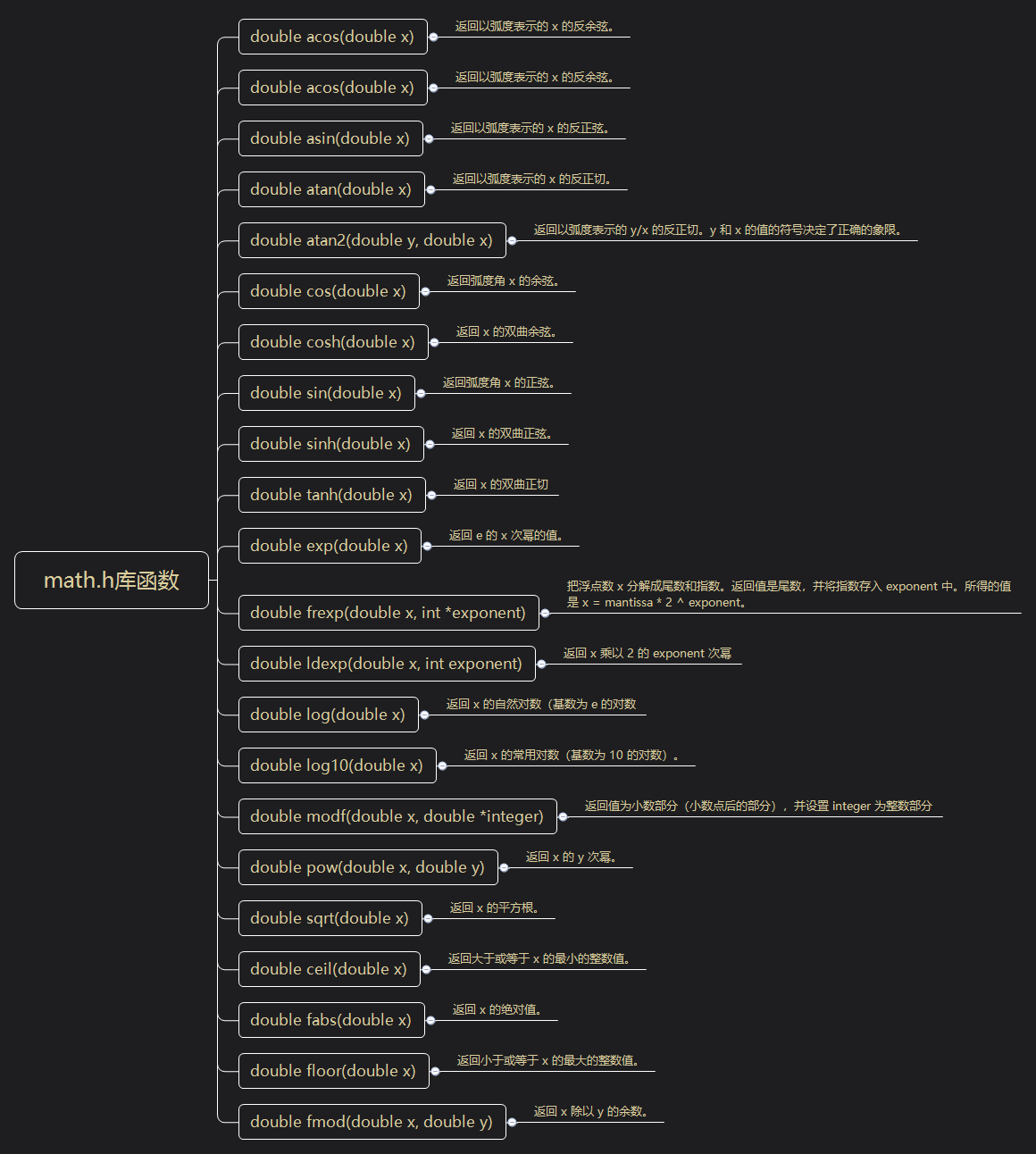前言
上回总结了string.h常用的字符串操作函数，这次总结一下另外的一个库函数math.h和数字运算有关联的库。
double acos(double x) 函数原型：double acos(double x) 功能：返回以弧度表示的 x 的反余弦。arcos(x);

//时间：2019年11月15日
//作者：Kroner
//编译环境：VS 2019
//库函数	 math.h
//函数原型   double acos(double x)
//函数功能： 返回以弧度表示的 x 的反余弦。
#define PI 3.14159265
#include "stdlib.h"
#include "stdio.h"
#include "math.h"
int main()
{
double x, ret, val;
x = 0.9;
val = 180.0 / PI;
ret = acos(x) * val;
printf("%lf 的反余弦是 %lf 度", x, ret);
return(0);
}


测试结果：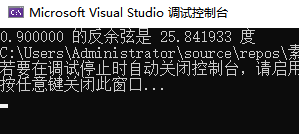double asin(double x) 函数原型：double asin(double x) 功能：返回以弧度表示的 x 的反余弦。arsin(x);

//时间：2019年11月15日
//作者：Kroner
//编译环境：VS 2019
//库函数	 math.h
//函数原型   double asin(double x)
//函数功能： 返回以弧度表示的 x 的反正弦。
#define PI 3.14159265
#include "stdlib.h"
#include "stdio.h"
#include "math.h"
int main()
{
double x, ret, val;
x = 0.9;
val = 180.0 / PI;

ret = asin(x) * val;
printf("%lf 的反正弦是 %lf 度", x, ret);

return(0);
}


测试结果：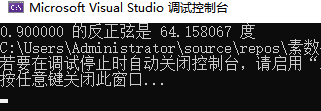double atan(double x) 函数原型：double atan(double x) 功能：返回以弧度表示的 x 的反正切。atan()

//时间：2019年11月15日
//作者：Kroner
//编译环境：VS 2019
//库函数	 math.h
//函数原型    double atan(double x)
//函数功能： 返回以弧度表示的 x 的反正切,atan()
#define PI 3.14159265
#include "stdlib.h"
#include "stdio.h"
#include "math.h"
int main()
{
double x, ret, val;
x = 1.0;
val = 180.0 / PI;
ret = atan(x) * val;
printf("%lf 的反正切是 %lf 度", x, ret);

return(0);
}

测试结果：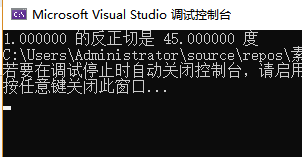double atan2(double y, double x) 函数原型 double atan2(double y, double x) 函数功能： C 库函数 double atan2(double y, double x) 返回以弧度表示的 y/x 的反正切。y 和 x 的值的符号决定了正确的象限。

//时间：2019年11月15日
//作者：Kroner
//编译环境：VS 2019
//库函数	 math.h
//函数原型    double atan2(double y, double x)
//函数功能： C 库函数 double atan2(double y, double x) 返回以弧度表示的 y/x 的反正切。y 和 x 的值的符号决定了正确的象限。
#define PI 3.14159265
#include "stdlib.h"
#include "stdio.h"
#include "math.h"

int main()
{
double x, y, ret, val;

x = -7.0;
y =  7.0;
val = 180.0 / PI;

ret = atan2(y, x) * val;
printf("x = %lf, y = %lf 的反正切", x, y);
printf("是 %lf 度\n", ret);

return(0);
}

测试结果：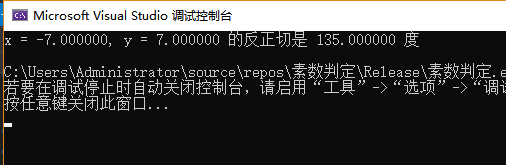double cos(double x) 函数原型： double cos(double x) 函数功能：返回弧度角 x 的余弦。

//时间：2019年11月15日
//作者：Kroner
//编译环境：VS 2019
//库函数	 math.h
//函数原型   double cos(double x)
//函数功能：返回弧度角 x 的余弦。
#define PI 3.14159265
#include "stdlib.h"
#include "stdio.h"
#include "math.h"

int main()
{
double x, ret, val;

x = 60.0;
val = PI / 180.0;
ret = cos(x * val);
printf("%lf 的余弦是 %lf 度\n", x, ret);

x = 90.0;
val = PI / 180.0;
ret = cos(x * val);
printf("%lf 的余弦是 %lf 度\n", x, ret);

return(0);
}

测试结果：double cosh(double x) 函数原型： double cosh(double x) 函数功能： 返回 x 的双曲余弦。
//时间：2019年11月15日
//作者：Kroner
//编译环境： VS 2019
//库函数	 math.h
//函数原型： double cosh(double x)
//函数功能： 返回 x 的双曲余弦。
#define PI 3.14159265
#include "stdlib.h"
#include "stdio.h"
#include "math.h"

int main()
{
double x;

x = 0.5;
printf("%lf 的双曲余弦是 %lf\n", x, cosh(x));

x = 1.0;
printf("%lf 的双曲余弦是 %lf\n", x, cosh(x));

x = 1.5;
printf("%lf 的双曲余弦是 %lf\n", x, cosh(x));

return(0);
}

测试结果：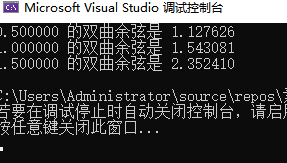double sin(double x) 函数原型： double sin(double x) 函数功能： 函数返回 x 的正弦。

//时间：2019年11月15日
//作者：Kroner
//编译环境： VS 2019
//库函数	 math.h
//函数原型： double sin(double x)
//函数功能： 函数返回 x 的正弦。
#define PI 3.14159265
#include "stdlib.h"
#include "stdio.h"
#include "math.h"

int main()
{
double x, ret, val;

x = 45.0;
val = PI / 180;
ret = sin(x * val);
printf("%lf 的正弦是 %lf 度", x, ret);

return(0);
}

测试结果：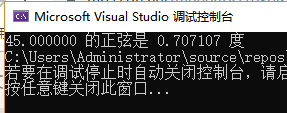double sinh(double x) 函数原型： double sinh(double x) 函数功能： 返回 x 的双曲正弦。

//时间：2019年11月15日
//作者：Kroner
//编译环境： VS 2019
//库函数	 math.h
//函数原型： double sinh(double x)
//函数功能： 返回 x 的双曲正弦。
#include <stdio.h>
#include <math.h>

int main()
{
double x, ret;
x = 0.5;

ret = sinh(x);
printf("%lf 的双曲正弦是 %lf 度", x, ret);

return(0);
}

测试结果：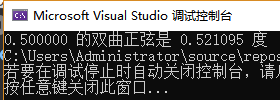double tanh(double x) 函数原型： double tanh(double x) 函数功能： 返回 x 的双曲正切。
//时间：2019年11月15日
//作者：Kroner
//编译环境： VS 2019
//库函数	 math.h
//函数原型： double tanh(double x)
//函数功能： 返回 x 的双曲正切。
#include <stdio.h>
#include <math.h>

int main()
{
double x, ret;
x = 0.5;

ret = tanh(x);
printf("%lf 的双曲正切是 %lf 度", x, ret);

return 0;
}

测试结果：double exp(double x) 函数原型： double exp(double x) 函数功能： 返回 e 的 x 次幂的值。
//时间：2019年11月15日
//作者：Kroner
//编译环境： VS 2019
//库函数	 math.h
//函数原型： double exp(double x)
//函数功能： 返回 e 的 x 次幂的值。
#include <stdio.h>
#include <math.h>

int main()
{
double x = 0;
printf("e 的 %lf 次幂是 %lf\n", x, exp(x));
printf("e 的 %lf 次幂是 %lf\n", x + 1, exp(x + 1));
printf("e 的 %lf 次幂是 %lf\n", x + 2, exp(x + 2));
return 0;
}

测试结果：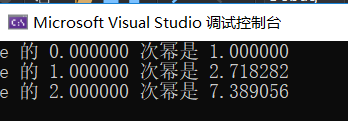double frexp(double x, int *exponent) 函数原型： double frexp(double x, int *exponent) 函数功能： 把浮点数 x 分解成尾数和指数。返回值是尾数，并将指数存入 exponent 中。所得的值是 x = mantissa * 2 ^ exponent。 详细说明：该函数返回规格化小数。如果参数 x 不为零，则规格化小数是 x 的二次方，且它的绝对值范围从 1/2（包含）到 1（不包含）。如果 x 为零，则规格化小数是零，且零存储在 exp 中。
//时间：2019年11月15日
//作者：Kroner
//编译环境： VS 2019
//库函数	 math.h
//函数原型： double frexp(double x, int *exponent)
//函数功能： 把浮点数 x 分解成尾数和指数。返回值是尾数，并将指数存入 exponent 中。所得的值是 x = mantissa * 2 ^ exponent。。
#include <stdio.h>
#include <math.h>

int main()
{
double x = 1024, fraction;
int e;

fraction = frexp(x, &e);
printf("x = %.2lf = %.2lf * 2^%d\n", x, fraction, e);

return 0;
}

测试结果：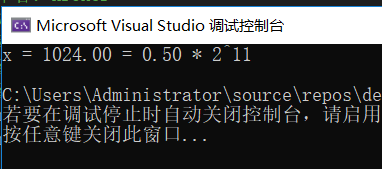double ldexp(double x, int exponent) 函数原型： double ldexp(double x, int exponent) 函数功能： 返回 x 乘以 2 的 exponent 次幂。返回 x * 2 exp。
//时间：2019年11月15日
//作者：Kroner
//编译环境： VS 2019
//库函数	 math.h
//函数原型： double ldexp(double x, int exponent)
//函数功能： 返回 x 乘以 2 的 exponent 次幂。
#include <stdio.h>
#include <math.h>

int main()
{
double x, ret;
int n;

x = 0.65;
n = 3;
ret = ldexp(x, n);
printf("%f * 2^%d = %f\n", x, n, ret);

return(0);
}

测试结果：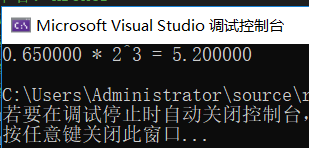double log(double x) 函数原型： double log(double x) 函数功能： 返回 x 的自然对数（基数为 e 的对数）
//时间：2019年11月15日
//作者：Kroner
//编译环境： VS 2019
//库函数	 math.h
//函数原型： double log(double x)
//函数功能： 返回 x 的自然对数（基数为 e 的对数）

#include <stdio.h>
#include <math.h>

int main()
{
double x, ret;
x = 2.7;

/* 计算 log(2.7) */
ret = log(x);
printf("log(%lf) = %lf", x, ret);

return 0;
}

测试结果：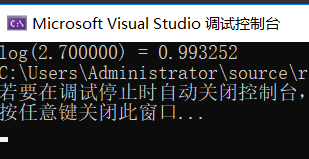double modf(double x, double *integer) 函数原型： double modf(double x, double *integer) 函数功能： 返回值为小数部分（小数点后的部分），并设置 integer 为整数部分。
//时间：2019年11月15日
//作者：Kroner
//编译环境： VS 2019
//库函数	 math.h
//函数原型： double modf(double x, double *integer)
//函数功能： 返回值为小数部分（小数点后的部分），并设置 integer 为整数部分。

#include <stdio.h>
#include <math.h>

#include<stdio.h>
#include<math.h>

int main()
{
double x, fractpart, intpart;

x = 8.123456;
fractpart = modf(x, &intpart);

printf("整数部分 = %lf\n", intpart);
printf("小数部分 = %lf \n", fractpart);

return 0;
}

测试结果：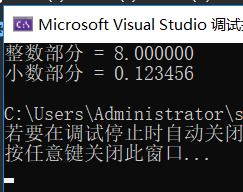double pow(double x, double y) 函数原型： double pow(double x, double y) 函数功能： 返回 x 的 y 次幂，即 x的y平方
//时间：2019年11月15日
//作者：Kroner
//编译环境： VS 2019
//库函数	  math.h
//函数原型：  double pow(double x, double y)
//函数功能：  返回 x 的 y 次幂，即 x的y平方
#include <stdio.h>
#include <math.h>

int main()
{
printf("值 8.0 ^ 3 = %lf\n", pow(8.0, 3));
printf("值 3.05 ^ 1.98 = %lf", pow(3.05, 1.98));
return 0;
}

测试结果：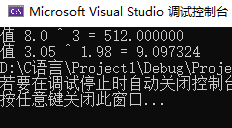double sqrt(double x) 函数原型： double sqrt(double x) 函数功能： 该函数返回 x 的平方根
//时间：2019年11月15日
//作者：Kroner
//编译环境： VS 2019
//库函数	 math.h
//函数原型：  double sqrt(double x)
//函数功能：  该函数返回 x 的平方根
#include <stdio.h>
#include <math.h>

int main()
{
printf("%lf 的平方根是 %lf\n", 4.0, sqrt(4.0));
printf("%lf 的平方根是 %lf\n", 5.0, sqrt(5.0));

return 0;
}

测试结果：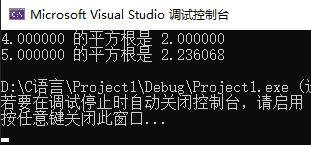double ceil(double x) 函数原型： double ceil(double x)  函数功能： 该函数返回不小于 x 的最小整数值
//时间：2019年11月15日
//作者：Kroner
//编译环境：  VS 2019
//库函数	  math.h
//函数原型：  double ceil(double x)
//函数功能：  该函数返回不小于 x 的最小整数值，可以理解为近1法
#include <stdio.h>
#include <math.h>

int main()
{
float val1, val2, val3, val4;

val1 = 1.6;
val2 = 1.2;
val3 = 2.8;
val4 = 2.3;

printf("value1 = %.1lf\n", ceil(val1));
printf("value2 = %.1lf\n", ceil(val2));
printf("value3 = %.1lf\n", ceil(val3));
printf("value4 = %.1lf\n", ceil(val4));

return 0;
}

测试结果：double fabs(double x) 函数原型： double fabs(double x) 函数功能： 返回 x 的绝对值。
//时间：2019年11月15日
//作者：Kroner
//编译环境：  VS 2019
//库函数	  math.h
//函数原型：  double fabs(double x)
//函数功能：  返回 x 的绝对值。

#include <stdio.h>
#include <math.h>

int main()
{
int a, b;
a = 1234;
b = -344;

printf("%d 的绝对值是 %lf\n", a, fabs(a));
printf("%d 的绝对值是 %lf\n", b, fabs(b));

return  0;
}

测试结果：double floor(double x) 函数原型： double floor(double x) 函数功能： 该函数返回不大于 x 的最大整数值。
//时间：2019年11月15日
//作者：Kroner
//编译环境：  VS 2019
//库函数	  math.h
//函数原型：  double floor(double x)
//函数功能：  该函数返回不大于 x 的最大整数值，可以理解为去尾巴

#include <stdio.h>
#include <math.h>

int main()
{
float val1, val2, val3, val4;
val1 = 1.6;
val2 = 1.2;
val3 = 2.8;
val4 = 2.3;

printf("Value1 = %.1lf\n", floor(val1));
printf("Value2 = %.1lf\n", floor(val2));
printf("Value3 = %.1lf\n", floor(val3));
printf("Value4 = %.1lf\n", floor(val4));
return 0;
}

测试结果：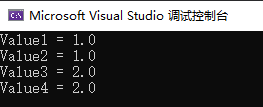double fmod(double x, double y) 函数原型： double fmod(double x, double y) 函数功能： 返回 x 除以 y 的余数。
//时间：2019年11月15日
//作者：Kroner
//编译环境：  VS 2019
//库函数	  math.h
//函数原型：  double fmod(double x, double y)
//函数功能：  返回 x 除以 y 的余数。

#include <stdio.h>
#include <math.h>

int main()
{
float a, b;
int c;
a = 9.2;
b = 3.7;
c = 2;
printf("%f / %d 的余数是 %lf\n", a, c, fmod(a, c));
printf("%f / %f 的余数是 %lf\n", a, b, fmod(a, b));

return 0;
}


展开全文C语言 常用函数库
• ## python math模块详解

千次阅读 多人点赞 2019-10-13 20:06:12
math模块主要包括数论与表示函数、幂函数与对数函数、三角函数、双曲函数、特殊函数，还有常量。还有math模块的一些小技巧
math — Mathematical functions

文章目录
math — Mathematical functions数论与表示函数幂函数与对数函数三角函数角度转换双曲函数特殊函数常量Math skill1. average - 平均值2. average_by - 函数映射后的平均值3. clamp_number4. digitize - 转数组5. factorial - 阶乘6. fibonacci - 斐波那契数列7. gcd - 最大公约数8. in_range - 判断范围9. is_divisible - 整除10. is_even - 偶数11. is_odd - 奇数12. 最小公倍数13. max_by - 函数映射后的最大值14. median - 中值15. min_by - 函数映射后的最小值16. rads_to_degrees - 弧度转角度17. sum_by - 求和

数论与表示函数
math.ceil(x) 返回 x 的向上取整，即大于或者等于 x 的最小整数。 如果 x 不是一个浮点数，则委托 x.__ceil__(), 返回 Integral 类的值。  math.copysign(x, y) 返回一个基于 x 的绝对值和 y 的符号的浮点数。 copysign(1.0, -0.0) 返回 -1.0.  math.fabs(x) 返回 x 的绝对值。  math.factorial(x) 以一个整数返回 x 的阶乘。 如果 x 不是整数或为负数时则将引发 ValueError。  math.floor(x) 返回 x 的向下取整，小于或等于 x 的最大整数。 如果 x 不是浮点数，则委托 x.__floor__() ，它应返回 Integral 值。  math.fmod(x, y) 返回 fmod(x, y) ，由平台C库定义。请注意，Python表达式 x % y 可能不会返回相同的结果。C标准的目的是 fmod(x, y) 完全（数学上；到无限精度）等于 x - n*y 对于某个整数 n ，使得结果具有 与 x 相同的符号和小于 abs(y) 的幅度。Python的 x % y 返回带有 y 符号的结果，并且可能不能完全计算浮点参数。 例如， fmod(-1e-100, 1e100) 是 -1e-100 ，但Python的 -1e-100 % 1e100 的结果是 1e100-1e-100 ，它不能完全表示为浮点数，并且取整为令人惊讶的 1e100 。 出于这个原因，函数 fmod() 在使用浮点数时通常是首选，而Python的 x % y 在使用整数时是首选。  math.frexp(x) 返回 x 的尾数和指数作为对(m, e)。 m 是一个浮点数， e 是一个整数，正好是 x == m * 2**e 。 如果 x 为零，则返回 (0.0, 0) ，否则返回 0.5 <= abs(m) < 1 。 这用于以可移植方式“分离”浮点数的内部表示。  math.fsum(iterable) 返回迭代中的精确浮点值。通过跟踪多个中间部分和来避免精度损失 >>> sum([.1, .1, .1, .1, .1, .1, .1, .1, .1, .1])
0.9999999999999999
>>> fsum([.1, .1, .1, .1, .1, .1, .1, .1, .1, .1])
1.0
  math.gcd(a, b) 返回整数 a 和 b 的最大公约数。如果 a 或 b 之一非零，则 gcd(a, b) 的值是能同时整除 a 和 b 的最大正整数。gcd(0, 0) 返回 0。  math.isclose(a, b, *, rel_tol=1e-09, abs_tol=0.0) 若 a 和 b 的值比较接近则返回 True，否则返回 False。 根据给定的绝对和相对容差确定两个值是否被认为是接近的。rel_tol 是相对容差 —— 它是 a 和 b 之间允许的最大差值，相对于 a 或 b 的较大绝对值。 例如，要设置5％的容差，请传递 rel_tol=0.05 。默认容差为 1e-09，确保两个值在大约9位十进制数字内相同。 rel_tol 必须大于零。abs_tol 是最小绝对容差 —— 对于接近零的比较很有用。 abs_tol 必须至少为零。  math.isfinite(x) 如果 x 既不是无穷大也不是NaN，则返回 True ，否则返回 False 。  math.isinf(x) 如果 x 是正或负无穷大，则返回 True ，否则返回 False 。  math.isnan(x) 如果 x 是 NaN（不是数字），则返回 True ，否则返回 False 。  math.ldexp(x, i) 返回 x * (2**i) 。 这基本上是函数 frexp()的反函数。  math.modf(x) 返回 x 的小数和整数部分。两个结果都带有 x 的符号并且是浮点数。  math.remainder(x, y) 返回 IEEE 754 风格的 x 相对于 y 的余数。对于有限 x 和有限非零 y ，这是差异 x - n*y ，其中 n 是与商 x /y 的精确值最接近的整数。如果 x / y 恰好位于两个连续整数之间，则最近的 * even* 整数用于 n 。 余数 r =remainder(x, y) 因此总是满足 abs(r) <= 0.5 * abs(y) 。 特殊情况遵循IEEE 754：特别是 remainder(x, math.inf) 对于任何有限 x 都是 x ，而 remainder(x, 0) 和 remainder(math.inf, x) 引发 ValueError 适用于任何非NaN的 x 。如果余数运算的结果为零，则该零将具有与 x 相同的符号。 在使用IEEE 754二进制浮点的平台上，此操作的结果始终可以完全表示：不会引入舍入错误。3.7 新版功能.  math.trunc(x) 返回 Real 值 x 截断为 Integral（通常是整数）。 委托给x.__trunc__()。
幂函数与对数函数
math.exp(x) 返回 e 次 x 幂，其中 e = 2.718281… 是自然对数的基数。 这通常比 math.e ** x 或 pow(math.e, x) 更精确。  math.expm1(x) 返回 e 的 x 次幂，减1。这里 e 是自然对数的基数。 对于小浮点数 x ， exp(x) - 1 中的减法可能导致 significant loss of precision；  math.log(x[, base]) 使用一个参数，返回 x 的自然对数（底为 e ）。 使用两个参数，返回给定的 base 的对数 x ，计算为 log(x)/log(base) 。  math.log1p(x) 返回 1+x (base e) 的自然对数。以对于接近零的 x 精确的方式计算结果。  math.log2(x) 返回 x 以2为底的对数。这通常比 log(x, 2) 更准确。  math.log10(x) 返回 x 底为10的对数。这通常比 log(x, 10) 更准确。  math.pow(x, y) 将返回 x 的 y 次幂。 特别是， pow(1.0, x) 和 pow(x, 0.0) 总是返回 1.0 ，即使 x 是零或NaN。 如果 x 和 y 都是有限的， x 是负数， y 不是整数那么 pow(x, y) 是未定义的，并且引发 ValueError。 与内置的 ** 运算符不同， math.pow()将其参数转换为 float类型。使用 ** 或内置的 pow() 函数来计算精确的整数幂。  math.sqrt(x) 返回 x 的平方根。
三角函数
math.acos(x) 以弧度为单位返回 x 的反余弦值。  math.asin(x) 以弧度为单位返回 x 的反正弦值。  math.atan(x) 以弧度为单位返回 x 的反正切值。  math.atan2(y, x) 以弧度为单位返回 atan(y / x) 。结果是在 -pi 和 pi 之间。 从原点到点 (x, y) 的平面矢量使该角度与正X轴成正比。 atan2() 的点的两个输入的符号都是已知的，因此它可以计算角度的正确象限。 例如， atan(1) 和 atan2(1, 1) 都是 pi/4 ，但 atan2(-1, -1) 是 -3*pi/4 。  math.cos(x) 返回 x 弧度的余弦值。  math.hypot(x, y) 返回欧几里德范数， sqrt(x*x + y*y) 。 这是从原点到点 (x, y) 的向量长度。  math.sin(x) 返回 x 弧度的正弦值。  math.tan(x) 返回 x 弧度的正切值。
角度转换
math.degrees(x) 将角度 x 从弧度转换为度数。  math.radians(x) 将角度 x 从度数转换为弧度。
双曲函数
双曲函数 是基于双曲线而非圆来对三角函数进行模拟。
math.acosh(x) 返回 x 的反双曲余弦值。  math.asinh(x) 返回 x 的反双曲正弦值。  math.atanh(x) 返回 x 的反双曲正切值。  math.cosh(x) 返回 x 的双曲余弦值。  math.sinh(x) 返回 x 的双曲正弦值。  math.tanh(x) 返回 x 的双曲正切值。
特殊函数
math.erf(x) 返回 x 处的 error function 。erf() 函数可用于计算传统的统计函数。  math.erfc(x) 返回 x 处的互补误差函数。 互补错误函数 定义为 1.0 - erf(x)。 它用于 x 的大值，从其中减去一个会导致 有效位数损失。  math.gamma(x) 返回 x 处的 伽马函数 值。  math.lgamma(x) 返回Gamma函数在 x 绝对值的自然对数。
常量
math.pi 数学常数 π = 3.141592…，精确到可用精度。  math.e 数学常数 e = 2.718281…，精确到可用精度。  math.tau 数学常数 τ = 6.283185…，精确到可用精度。 Tau 是一个圆周常数，等于 2π，圆的周长与半径之比。  math.inf 浮点正无穷大。 （对于负无穷大，使用 -math.inf 。）相当于float('inf') 的输出。  math.nan 浮点“非数字”（NaN）值。 相当于 float('nan') 的输出。
Math skill
1. average - 平均值
返回两个或多个值的平均值
Returns the average of two or more numbers.
Use sum() to sum all of the args provided, divide by len(args).
def average(*args):
return sum(args, 0.0) / len(args)
 Examples
average(*[1, 2, 3]) # 2.0
average(1, 2, 3) # 2.0

2. average_by - 函数映射后的平均值
返回一个列表中所有经过函数处理的元素的平均值
Returns the average of a list, after mapping each element to a value using the provided function.
Use map() to map each element to the value returned by fn. Use sum() to sum all of the mapped values, divide by len(lst).
def average_by(lst, fn=lambda x: x):
return sum(map(fn, lst), 0.0) / len(lst)
 Examples
average_by([{ 'n': 4 }, { 'n': 2 }, { 'n': 8 }, { 'n': 6 }], lambda x: x['n']) # 5.0

3. clamp_number
将num限制在边界值a和b指定的范围内。
如果num在此范围内，则返回num。
否则，返回范围内最接近的数字。
Clamps num within the inclusive range specified by the boundary values a and b.
If num falls within the range, return num. Otherwise, return the nearest number in the range.
def clamp_number(num,a,b):
return max(min(num, max(a,b)),min(a,b))
 Examples
clamp_number(2, 3, 5) # 3
clamp_number(1, -1, -5) # -1

4. digitize - 转数组
将一个数转换为数字数组。
Converts a number to an array of digits.
Use map() combined with int on the string representation of n and return a list from the result.
def digitize(n):
return list(map(int, str(n)))
 Examples
digitize(123) # [1, 2, 3]

5. factorial - 阶乘
计算数字的阶乘
Calculates the factorial of a number.
Use recursion. If num is less than or equal to 1, return 1. Otherwise, return the product of num and the factorial of num - 1. Throws an exception if num is a negative or a floating point number.
def factorial(num):
if not ((num >= 0) and (num % 1 == 0)):
raise Exception(
f"Number( {num} ) can't be floating point or negative ")
return 1 if num == 0 else num * factorial(num - 1)
 Examples
factorial(6) # 720

6. fibonacci - 斐波那契数列
生成斐波那契数列
Generates an array, containing the Fibonacci sequence, up until the nth term.
Starting with 0 and 1, use list.apoend() to add the sum of the last two numbers of the list to the end of the list, until the length of the list reaches n. If n is less or equal to 0, return a list containing 0.
def fibonacci(n):
if n <= 0:
return 

sequence = [0, 1]
while len(sequence) <= n:
next_value = sequence[len(sequence) - 1] + sequence[len(sequence) - 2]
sequence.append(next_value)

return sequence
 Examples
fibonacci(7) # [0, 1, 1, 2, 3, 5, 8, 13]

7. gcd - 最大公约数
计算数字列表的最大公约数。
Calculates the greatest common divisor of a list of numbers.
Use reduce() and math.gcd over the given list.
from functools import reduce
import math

def gcd(numbers):
return reduce(math.gcd, numbers)
 Examples
gcd([8,36,28]) # 4

8. in_range - 判断范围
检查给定数字是否在给定范围内
Checks if the given number falls within the given range.
Use arithmetic comparison to check if the given number is in the specified range. If the second parameter, end, is not specified, the range is considered to be from 0 to start.
def in_range(n, start, end = 0):
if (start > end):
end, start = start, end
return start <= n <= end
 Examples
in_range(3, 2, 5); # True
in_range(3, 4); # True
in_range(2, 3, 5); # False
in_range(3, 2); # False

9. is_divisible - 整除
检查第一个数值参数是否可被第二个数值参数整除。
Checks if the first numeric argument is divisible by the second one.
Use the modulo operator (%) to check if the remainder is equal to 0.
def is_divisible(dividend, divisor):
return dividend % divisor == 0
 Examples
is_divisible(6, 3) # True

10. is_even - 偶数
如果给定数字为偶数，则返回’true’，否则返回’false’。
Returns True if the given number is even, False otherwise.
Checks whether a number is odd or even using the modulo (%) operator. Returns True if the number is even, False if the number is odd.
def is_even(num):
return num % 2 == 0
 Examples
is_even(3) # False

11. is_odd - 奇数
如果给定数字为偶数，则返回’true’，否则返回’false’。
Returns True if the given number is odd, False otherwise.
Checks whether a number is even or odd using the modulo (%) operator. Returns True if the number is odd, False if the number is even.
def is_odd(num):
return num % 2 != 0
 Examples
is_odd(3) # True

12. 最小公倍数
返回两个或多个数字的最小公倍数。
Returns the least common multiple of two or more numbers.
Define a function, spread, that uses either list.extend() or list.append() on each element in a list to flatten it. Use math.gcd() and lcm(x,y) = x * y / gcd(x,y) to determine the least common multiple.
from functools import reduce
import math

ret = []
for i in arg:
if isinstance(i, list):
ret.extend(i)
else:
ret.append(i)
return ret

def lcm(*args):
numbers = []

def _lcm(x, y):
return int(x * y / math.gcd(x, y))

return reduce((lambda x, y: _lcm(x, y)), numbers)
 Examples
lcm(12, 7) # 84
lcm([1, 3, 4], 5) # 60

13. max_by - 函数映射后的最大值
在使用所提供的函数将每个元素映射到每一个值后返回一个列表的最大值。
Returns the maximum value of a list, after mapping each element to a value using the provided function.
Use map() with fn to map each element to a value using the provided function, use max() to return the maximum value.
def max_by(lst, fn):
return max(map(fn,lst))
 Examples
max_by([{ 'n': 4 }, { 'n': 2 }, { 'n': 8 }, { 'n': 6 }], lambda v : v['n']) # 8

14. median - 中值
查找列表中元素的中值。
Finds the median of a list of numbers.
Sort the numbers of the list using list.sort() and find the median, which is either the middle element of the list if the list length is odd or the average of the two middle elements if the list length is even.
def median(list):
list.sort()
list_length = len(list)
if list_length%2==0:
return (list[int(list_length/2)-1] + list[int(list_length/2)])/2
else:
return list[int(list_length/2)]
 Examples
median([1,2,3]) # 2
median([1,2,3,4]) # 2.5

15. min_by - 函数映射后的最小值
在使用所提供的函数将每个元素映射到每一个值后返回一个列表的最小值。
Returns the minimum value of a list, after mapping each element to a value using the provided function.
Use map() with fn to map each element to a value using the provided function, use min() to return the minimum value.
def min_by(lst, fn):
return min(map(fn,lst))
 Examples
min_by([{ 'n': 4 }, { 'n': 2 }, { 'n': 8 }, { 'n': 6 }], lambda v : v['n']) # 2

将角度从弧度转换为角度。
Converts an angle from radians to degrees.
Use math.pi and the radian to degree formula to convert the angle from radians to degrees.
import math

return (rad * 180.0) / math.pi
 Examples
import math

17. sum_by - 求和
使用提供的函数将每个元素映射到值后，返回列表的和。
Returns the sum of a list, after mapping each element to a value using the provided function.
Use map() with fn to map each element to a value using the provided function, use sum() to return the sum of the values.
def sum_by(lst, fn):
return sum(map(fn,lst))
 Examples
sum_by([{ 'n': 4 }, { 'n': 2 }, { 'n': 8 }, { 'n': 6 }], lambda v : v['n']) # 20

展开全文• Apache Commons Math下的jar包commons-math3-3.0.jar
• Apache Common Math 3.2 API CHM 英文文档。 注意：如果你下载后打开右侧页面出现该页无法显示情况，请在CHM件中右键-属性，在下面点击-解除锁定。之后再打开文档即可。
• ## MathML 与 Latex 互转

热门讨论 2014-03-07 16:40:22
$\sum_{i=1}^n a_i=0$ Latex 与 MathML 互转
• ## mathpix替代品终于找到了

万次阅读 热门讨论 2019-10-04 19:16:44
• ## Mathpix教程

万次阅读 多人点赞 2019-11-29 15:46:38
• Doing Math with Python shows you how to use Python to delve into high school—level math topics like statistics, geometry, probability, and calculus. You'll start with simple projects, like a ...
• 文章目录Python 标准库之 Math 数学函数导入模块Math 常量math.pimath.emath.taumath.infmath.nanmath.ceil(x)math.floor(x)math.comb(n, k)math.copysign(x, y)math.fabs(x)math.factorial(x)math.fmod(x, y)math....python 数学
• ## math.h头文件

热门讨论 2012-11-27 21:32:59
编译时你可能会提示缺少一个头文件 这就是其中之一的头文件 尤其是很多数学运算会用到这个头文件
• Math是一个内置对象，它拥有一些数学常数属性和数学函数方法。Math 不是一个函数对象。Math 用于 Number 类型。 Math 不是一个构造器。Math 的所有属性与方法都是静态的。Math 的常量是使用 JavaScript 中的全精度...
• ## Java Math类方法介绍之史上最全

千次阅读 多人点赞 2019-05-11 17:32:13
Java Math类方法介绍之史上最全 开发中用到了Java Math类的方法，故温习记录如下。 /** * 常量 */ System.out.println("------>" + Math.E);//2.718281828459045 System.out.println("------>" + Math....Java android
• 相信大家都知道mathpix吧 一款特别的牛逼的img2latex软件 如果有小伙伴不知道的话可以看我之前的博客 超级利器——mathpix 文本、图片公式转md/latex格式 牛逼虽说是牛逼，但是对于普通的用户来说每个月只有50次免费...python
• ## Math类常用方法大全

万次阅读 多人点赞 2018-06-12 15:18:05
1.简介Java的Math类封装了很多与数学有关的属性和方法。2.举例说明代码：private void mathMethod(){ /** * Math.sqrt()//计算平方根 * Math.cbrt()//计算立方根 * Math.hypot(x,y)//计算 (x的平方+y的平方)的...
• .NET中的Math.Floor()和Math.Truncate()什么区别？.net
• ## Symbolic Math Toolbox

热门讨论 2012-10-20 14:29:48
Matlab Symbolic TOOlbox Matlab R2012bMatlab
• ## JAVA基础（Math类）

万次阅读 2019-06-16 17:59:41
1，Math类概述 类包含用于执行基本数学运算的方法 Math 类包含用于执行基本数学运算的方法，如初等指数、对数、平方根和三角函数。 Math所有类都是静态的。可以直接类名。调用。 2，成员方法 ...
• int b=(int)(Math.random()*10);//生成[0,9]之间的随机整数。 生成从n到m的随机整数 int temp=m+(int)(Math.random()*(n+1-m)); //生成从m到n的随机整数[m,n] 原文：java Math.random()生成从n到m的随机整数 ...Java随机数
• 深入学习java源码之Math.max()与 Math.min() java基本数据类型及自动转型 8种基本数据类型及其所占空间大小： 一、byte，占用一个字节，取值范围为 -128-127，默认是“\u0000”，表示空 二、short，占用两个字节...
• math类中提供了三个与取整有关的方法：ceil,floor,round,这些方法的作用与它们的英文名称的含义相对应。 例如： ceil的英文意义是天花板，该方法就表示向上取整，math.ceil（11.3）的结果为12，math.ceil(-11.6)的...java
• pygame.math pygame模块关于向量类 pygame.math.Vector2 - 一个二维向量 pygame.math.Vector3 - 一个三维向量 pygame.math.enable_swizzling - 全局允许对向量进行调配 pygame.math.disable_swizzling - 全局禁用对...Pygame 官方文档...